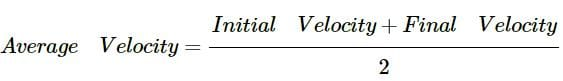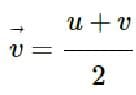Test: Acceleration

# Test: Acceleration - Class 9

Test Description

## 10 Questions MCQ Test Science Class 9 - Test: Acceleration

Test: Acceleration for Class 9 2023 is part of Science Class 9 preparation. The Test: Acceleration questions and answers have been prepared according to the Class 9 exam syllabus.The Test: Acceleration MCQs are made for Class 9 2023 Exam. Find important definitions, questions, notes, meanings, examples, exercises, MCQs and online tests for Test: Acceleration below.
Solutions of Test: Acceleration questions in English are available as part of our Science Class 9 for Class 9 & Test: Acceleration solutions in Hindi for Science Class 9 course. Download more important topics, notes, lectures and mock test series for Class 9 Exam by signing up for free. Attempt Test: Acceleration | 10 questions in 10 minutes | Mock test for Class 9 preparation | Free important questions MCQ to study Science Class 9 for Class 9 Exam | Download free PDF with solutions
 1 Crore+ students have signed up on EduRev. Have you?
Test: Acceleration - Question 1

### A bus decelerating with 4m/s2 changes its speed from 60 m s-1 to a certain value in 5 s. The final speed is:​

Detailed Solution for Test: Acceleration - Question 1

Acceleration = – 4m/s2; Initial speed, u = 60 m s-1

Time taken = 5s

Acceleration = change in velocity per unit time

a=v-u/t

v= u + at

v = 60 – 4 x 5 = 40 m/s

Test: Acceleration - Question 2

### If the upward direction is taken as positive, what is the sign of velocity and acceleration when a body falls freely from a height?

Detailed Solution for Test: Acceleration - Question 2

Both velocity and acceleration are in the downward direction. Thus, they will be considered negative as they are vectors and the upward direction is positive.

Test: Acceleration - Question 3

### A body moving along a straight line at 20m/s undergoes an acceleration of -4m/s2. After two seconds its speed will be______.

Detailed Solution for Test: Acceleration - Question 3

u = 20 m/s, a = -4 m/s2, t = 2 s.
v = ?
∵ v = u + at
⇒ v = 20 + (-4) (2)
⇒ v = 20 - 8 = 12 m/s

Test: Acceleration - Question 4

A boy throws a ball up and catches it when the ball falls back. In which part of the motion the ball is accelerating?

Detailed Solution for Test: Acceleration - Question 4
• When the ball moves upward, the force of gravitation is acting in the opposite direction of the ball that is why in that case acceleration is negative or in other words deceleration takes place.
• But when the ball is moving downwards the force of gravitation is in the same direction of the motion of the ball thus acceleration takes place in this case.
Test: Acceleration - Question 5

Choose the correct option:

Detailed Solution for Test: Acceleration - Question 5
• Distance is a scalar quantity that refers to "how much ground an object has covered" during its motion.
• Acceleration is a vector quantity that is defined as the rate at which an object changes its velocity.
• Velocity is a vector quantity that refers to the speed of an object in a particular direction.
Test: Acceleration - Question 6

The ratio of C.G.S. to M.K.S. unit of acceleration is:​

Detailed Solution for Test: Acceleration - Question 6

M.K.S. unit of acceleration = 1 m/s2
C.G.S. unit of acceleration = 1 cm/s2

C.G.S unit of acceleration/ M,K.S unit of acceleration = 1 cm/s2 / 1 m/s2
= 1 cm / 100 cm = 1/100 =1:100

Test: Acceleration - Question 7

The S.I. unit of acceleration is______.

Detailed Solution for Test: Acceleration - Question 7

Calculating acceleration involves dividing velocity by time or we can say,  dividing the meter per second by the second. i.e. [ m/s2 = (m/s) /s ]

Test: Acceleration - Question 8

If a moving body comes to rest, then its acceleration is______.

Detailed Solution for Test: Acceleration - Question 8

If a moving body comes to rest, then its acceleration is negative, as it's velocity becomes zero, therefore the acceleration is also zero.

Test: Acceleration - Question 9

If the average velocity of a body is equal to mean of its initial velocity and final velocity, then the acceleration of the body is:

Detailed Solution for Test: Acceleration - Question 9

If the velocity of the body is always changing with a uniform rate (uniform acceleration), then the average velocity is given byTest: Acceleration - Question 10

Acceleration is defined as the rate of change of______.

Detailed Solution for Test: Acceleration - Question 10

Acceleration is a vector quantity that is defined as the rate at which an object changes its velocity. An object is accelerating if it is changing its velocity.

## Science Class 9

66 videos|352 docs|97 tests